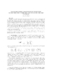## Backward Error Analysis of Factorization Algorithms for Symmetric and Symmetric Triadic Matrices##### Files
backward.pdf(195.87 KB)
We consider the $LBL^T$ factorization of a symmetric matrix where $L$ is unit lower triangular and $B$ is block diagonal with diagonal blocks of order $1$ or $2$. This is a generalization of the Cholesky factorization, and pivoting is incorporated for stability. However, the reliability of the Bunch-Kaufman pivoting strategy and Bunch's pivoting method for symmetric tridiagonal matrices could be questioned, because they may result in unbounded $L$. In this paper, we give a condition under which $LBL^T$ factorization will run to completion in inexact arithmetic with inertia preserved. In addition, we present a new proof of the componentwise backward stability of the factorization using the inner product formulation, giving a slight improvement of the bounds in Higham's proofs, which relied on the outer product formulation and normwise analysis. We also analyze the stability of rank estimation of symmetric indefinite matrices by $LBL^T$ factorization incorporated with the Bunch-Parlett pivoting strategy, generalizing results of Higham for the symmetric semidefinite case. We call a matrix triadic if it has no more than two non-zero off-diagonal elements in any column. A symmetric tridiagonal matrix is a special case. In this paper, we display the improvement in stability bounds when the matrix is triadic.